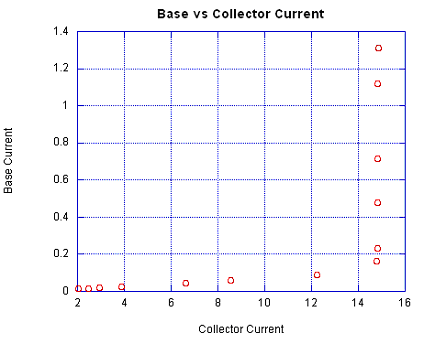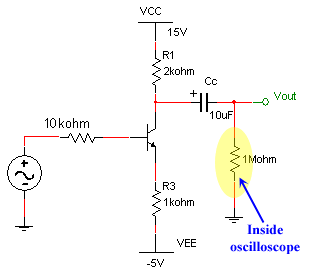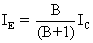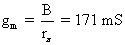# Engineering 72 Lab 4

## Bipolar Transistors

#### Base Current vs Collector CurrentWe measured the actual values of the supposed 10 k and 1 k resistor as 9.82 k and .992 k respectively. To calculate IC and IB, the measured voltage across the resistors was divided by the resistor’s respective resistance. From our calculated values of IC & IB, we found B to be approximately equal to 144.

 Voltage across 1k resistor Voltage across 10k resistor R IC IB B 12.89 14.74 913 1.313 14.859 11.320 11.01 14.72 2740 1.121 14.839 13.235 7.03 14.71 9830 0.716 14.829 20.714 4.69 14.71 19600 0.478 14.829 31.048 2.29 14.7 50400 0.233 14.819 63.545 1.61 14.67 75800 0.164 14.788 90.199 0.86 12.12 1.51E+05 0.088 12.218 139.510 0.58 8.49 2.27E+05 0.059 8.558 144.904 0.45 6.56 2.96E+05 0.046 6.613 144.308 0.26 3.87 5.13E+05 0.027 3.901 144.612 0.2 2.92 6.82E+05 0.020 2.944 144.528 0.17 2.46 8.07E+05 0.017 2.480 143.247 0.13 2.03 9.75E+05 0.013 2.046 154.580#### Part 2: Simple Common Emitter Amplifier

When performing the DC analysis, we neglect the AC input source and connect the input simply to ground. The resulting circuit is shown below. Note that the component values and voltages shown below are the measured resistances and measured voltages from the circuit used in the lab.We begin the analysis by computing the voltage at the base of the transistor, Vb.Thus, Vb = -0.2796 Volts. By dividing by the 9.57k resistor, we get a base current of 0.0292 mA, and consequently a collector current of 4.207 mA.

In the lab, the measured voltage across R2 (1.977k) was 8.32 V. Dividing by the resistance, we get a 'measured' collector current of 4.208 mA, which is essentially identical to the theoretical value calculated above.

Transistor amplifiers need to be biased so that the DC component of the input signal is centered on the linear part of the transistor voltage characteristic. Because of the steep slope of this section of the graph, the input voltage can swing only very little (usually a few tenths of a volt) before sending the output into either cutoff or saturation. In order to obtain the maximum undistorted positive and negative swing in the output voltage, it is necessary to bias the input to operate centered at this midpoint value of the linear region. However, if only a positive output voltage were required, the input would be biased not at the center but at the edge of the linear region to achieve the maximum positive output voltage swing possible without distortion.

#### Part 3: Comparison of DC Offsets

Given the collector current of 4.207 mA calculated in the DC analysis, we can determine the DC component of the output voltage. With AC signals at 0, the output voltage is given by 14.72 V - 1.977k * 4.207 mA = 6.402 V.

The oscilloscope output below shows the AC input signal (Ch1) and the amplifier output voltage (Ch2). The mean value of the output voltage is shown at 6.44 Volts, which is the DC component of the AC signal. This value is very close to the expected theoretical DC offset of 6.40 Volts.#### Part 4: Output Coupling CapacitorA capacitor acts like an open circuit at DC because the capacitor completely charges and prevents current from flowing. At AC with a high frequency (such as 10k Hertz), the capacitor is unable to significantly charge and acts like a short circuit. Therefore, the capacitor amplifies the AC signal without the DC offset.

The gain of the circuit (Avo)= Vout/Vin = -3.34/1.78 = -1.88.

For the following circuitthe capacitor acts like an open circuit at DC. Therefore, the DC model is represented byFor a npn transistor, IE=(B+1)IB. Solving for the loop shown aboveIE = 4.27 mAIC = 4.3 mA

Determining the small signal modelWe can now solve the gain of the circuitRc = RE = 2000 ohms
Avo = -1.86 This is very close to the measured gain of -1.88.

#### Part 5: Emitter BypassedWith an AC input, the 330uF capacitor acts like a short circuit and there is no voltage drop across the 1k ohm resistor. Therefore, summing the voltages as shown belowwe find that IB increases, thereby increasing IE. Because IC is linearly dependent on IE, IC increases. As IC increases, there is a greater drop across the 2k resistor and Vout increases.

The measured gain of the circuit (Avo)= Vout/Vin = -5.12/.228 = -22.46.

Calculated gain = -26. 57. This gain assumes an infinite capacitance. Since this is impossible, there is still a small impedance at the emitter, decreasing the actual gain of the circuit.

#### Part 6: Identifying Cutoff and Saturation Regions

A 1.47 V peak-to-peak input signal resulted in distorted output signal with a 11.9 V peak-to-peak. The upper distortion is caused when the transistor enters cutoff, and the lower distortion happens when the transistor enters saturation. When the transistor is in cutoff mode, no current flows, meaning that Vo is essentially pulled to Vcc. As a result, cutoff distortion will happen at the upper peak of the output signal.#### Part 7: A Better Voltage RegulatorThis circuit works far better than the simple zener regulator from lab 3 because the transistor essentially decouples the load's current drain from the zener diode's voltage regulation. The reverse biased diode maintains 6.2 volts across it as long as the current through it is large enough to stay away from the knee in the current-voltage characteristic. With the simple regulator from the previous lab, the load was placed in parallel with the zener diode, meaning that a small resistance load would drain a large current, causing the current through the zener diode to slip past the knee point, and as a result the voltage across the zener would drop. With the regulator circuit above, the emitter voltage is kept at a constant 0.7 V below the zener voltage, and a reduction in the load resistance (resulting in a larger current drain) would pull more current through the transistor and barely affect the current into the base. Thus, the zener voltage would remain constant.

To show this, we can replace the transistor with a linear model. The input resistance to the base of the transistor is given bywhere rL is the load resistance attached to the regulator. For a beta value of approximately 100, riwill only feel about 1/100th the change experienced in rL, meaning that the transistor will draw very little extra base current.

#### Part 8: The OpAmp-Based Voltage RegulatorThe expected output voltage Vout can readily be calculated by noting that the reverse-bias voltage of the zener diode is 6.2 Volts, on the assumption that sufficient current flows through it to stay clear of the knee point. A simple voltage divider calculation reveals that the positive terminal of the opamp is at 4.133 volts. Because the opamp attempts to push the two input terminals to the same voltage, the output voltage Vo, which is connected to the negative terminal, will also be driven to 4.133 volts.

The circuit works even better than the transistor-zener based voltage regulator presented in part 7 because the feedback system on the opamp essentially ensures that the base voltage of the transistor is raised high enough to allow enough current through to maintain the stable output voltage regardless of the attached load. Suppose that the load resistance is lowered such that more current is drawn. As a result, the output voltage temporarily drops, but since Vout is connected to the negative feedback of the opamp, the output voltage of the opamp will increase to counteract the change. More current will flow through the transistor, satisfying the current drain of the load, while maintaining an essentially constant output voltage.

The other notable feature of this circuit is that the output voltage of the regulator can easily be picked by choosing appropriate R2 and R3 values. Also, the 120 Ohm resistor can be replaced by a 240 Ohm resistor. This is possible since the current through the zener diode is unaffected by the load, meaning that less current needs to be pushed through the diode to ensure a constant voltage drop. Less current goes to waste as heat as a result.

The LM324 opamp is used in this configuration because the output voltage can move right up to the voltage of the supply rails, unlike the LM411 that could only get within approximately 2 Volts of the positive and negative supplies.

#### Part 9: Crossover DistortionThe crossover distortion can be attributed to the sinusoidal output of the Op Amp. When the output of the Op Amp is between -0.7 and 0.7 volts, the transistors are no longer in their active stages. No current flows and Vout is equal to zero.

#### Part 10: Circuit Modifications to Remove Cross-Over Distortion

To fix the crossover distortion in the output voltage, we applied the same idea used in the "superdiode" from Lab 3. By changing the feedback path of the circuit directly to the main output Vout instead of just the op-amp's intermediate output, the op-amp will drive the base of the transistors far higher during the crossover period to overcome the 0.7 volt transistor threshold as fast as possible. The speed with which the op amp can provide a nearly vertical voltage spike to overcome the threshold is limited by the op-amp's slew rate. In both the superdiode and the circuit below, we can ignore the non-linearities in the gain of the circuit as long as the feedback path is linear.#### Part 11: Output of Pumped Up Op AmpThe nearly vertical jumps in the opamp's output voltage are clearly seen on the channel 2 trace. These voltage spikes immediately overcome the 0.7 V diode drop in the transistor, and thus maintain the constant voltage at the output terminal of the regulator circuit. Also, since the feedback is now directly connected to the output, the amplitude of the input voltage to the opamp is maintained, as explained in the previous sections.